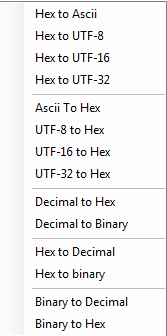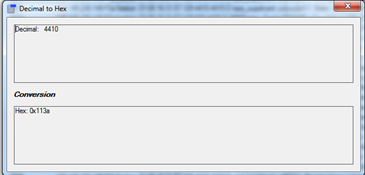#####Convert Text

This option opens the conversion menu with the following options:

Hex to Ascii/UTF-8/UTF-16/UTF-32: Convert hex numbers to corresponding asci characters.

Ascii/UTF-8/UTF-16/UTF-32 to Hex: Convert a string to Hex numbers

Decimal to Hexadecimal/Binary: Convert a decimal number to a hexadecimal or binary representation

Hexadecimal to Decimal/Binary: Convert a decimal number to a hexadecimal or binary representationBinary to Decimal/Hexadecimal: Convert a binary number to a decimal or hexadecimal representation